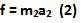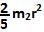Wednesday, December 17, 2008

Irodov Problem 1.261As soon as the plank is pulled forward, if there were no friction the sphere would have stayed exactly where it is while the plank moved undreneath it. There would be slipping between the sphere and the plank. The presence of friction however, cause a resistive force to avoid this slipping. The friction force acts in opposite directions on the palnk and the sphere. For the plank the force of friction f opposes force F, however for the sphere the friction force f induces the sphere to roll backwards relative to an observer on the plank (the net motion of the sphere as seen by the stationary observer will still be forward).
There are two forces acting on the Plank, i) the force F and the force of friction f. Let its acceleration be a1. So we have,There is only one force acting on the sphere - the force of friction f. Let the sphere's acceleration be a2. So we have,The friction force f also induces a torque on the sphere making it spin counter-clockwise. Let the angualr acceleration of the sphere be.If the radius of the sphere is r, the moment of inertia of the sphere about its axis of rotation is.Then we have,

The sphere rolls on the moving plank and so as seen by an observer on the plank its linear acceleration in the backward direction relative to the plank must be equal to its angular acceleration times its radius. So we have,Now we can solve for the accelerations of the plank and the sphere as,